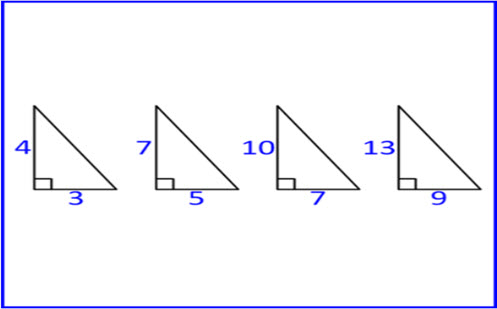Go to website

# Right-angled triangles inquiry

This prompt will guide students to make a connection between Pythagoras and trigonometrical ratios. This can build the students' conceptual understanding of what a trigonometrical ratio is. The resource includes classroom examples, teacher notes and guided questions.

Year level(s) Year 8, Year 9
Audience Teacher
Teaching strategies and pedagogical approaches Collaborative learning, Differentiated teaching, Explicit teaching, Feedback, Mathematics investigation
Keywords right-angled triangle, Pythagoras, trigonometry, ratio, similar, scale factor

## Curriculum alignment

Curriculum connections Critical and creative thinking, Numeracy
Strand and focus Measurement, Space, Build understanding, Apply understanding
Topics Pythagoras and trigonometry
AC: Mathematics (V9.0) content descriptions
AC9M8M06

Use Pythagoras' theorem to solve problems involving the side lengths of right-angled triangles

AC9M9SP02
Apply the enlargement transformation to shapes and objects using dynamic geometry software as appropriate; identify and explain aspects that remain the same and those that change

Numeracy progression Understanding units of measurement (P10)
Understanding geometric properties (P7)
Multiplicative strategies (P9)
Proportional thinking (P7)